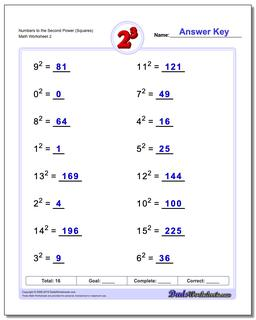Math Worksheets

### Worksheet News

PLEASE GO BACK AND USE THE BIG BLUE 'PRINT' BUTTON ON THE PAGE TO PRINT THE WORKSHEET CORRECTLY!Sorry for the trouble! The browser won't print the embedded worksheet PDF directly using the normal 'Print' command in the file menu, so you need to click the big 'Print' button to send just the worksheet and not the surrounding page to the printer.

# Math Worksheets: Exponents: Exponents: Numbers to the Second Power (Squares) (Second Worksheet)## Numbers to the Second Power (Squares) (Second Worksheet)

Property Value
DescriptionNumbers to the Second Power (Squares): Practice exponents worksheets introducing exponent syntax, calculation of simple exponents, powers of ten and scientific notation. The first of these exponents worksheets include hints and will build familiarity with many common exponential terms. (Second Worksheet)
Resource TypeWorksheet